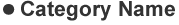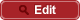• HOME
• RIKEN-QHP
• Search for $\Upsilon(1S,2S) \to Z^+_c Z^{(′)－}_c$ and $e^+ e^－ \to Z^+_c Z^{(′)－}_c$ at $\sqrt{s} = 10.52$, 10.58, and 10.867 GeV

##PrePrint No. RIKEN-QHP-389 S.Jia et al., (Belle Collaboration) including M. Takizawa Search for $\Upsilon(1S,2S) \to Z^+_c Z^{(′)－}_c$ and $e^+ e^－ \to Z^+_c Z^{(′)－}_c$ at $\sqrt{s} = 10.52$, 10.58, and 10.867 GeV arXiv: 1805.02308  (org) Phys. Rev. D97, 112004 (2018) 2018.05